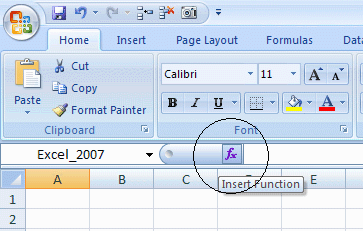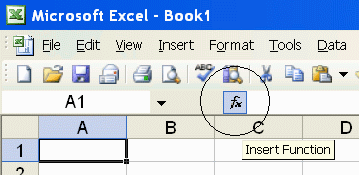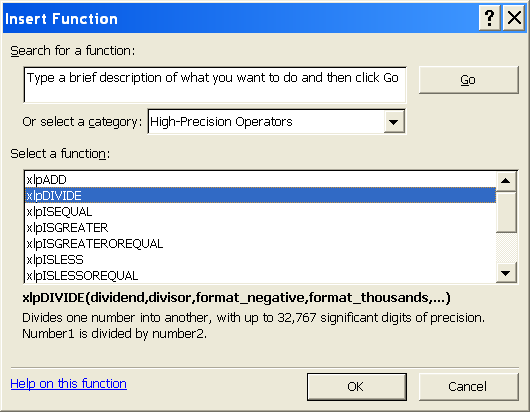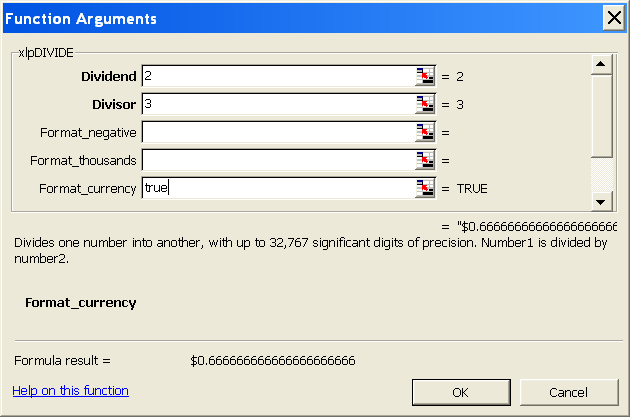PrecisionCalc
xl
Precision
Get Your Numbers Right

xlpBASECONV

Converts an integer to another number base, with up to 32,767 significant digits of precision.

The source number base and target number base can both be any base from base 2 through base 36.

Requires the 5,000 SD edition of xlPrecision or higher, or the Free Edition.

xlpBASECONV was introduced in xlPrecision 2017. It is not included in versions 3.x.x and earlier.

Syntax

xlpBASECONV(integer_num,from_base,to_base)

 integer_num Required. The number to be converted. Must be an integer.Cannot include digits that do not exist in from_base. For example, if from_base is 3, then integer_num must not include digits higher than 2. Use this standard set of 36 base digits: 0123456789ABCDEFGHIJKLMNOPQRSTUVWXYZ For example, for base 10 numbers, use only digits 0-9. For base 16 numbers, use only digits 0-F. Base 36 digits may use all 36 digits 0-Z. from_base Required. The number base of integer_num before conversion to to_base. Minimum from_base is 2. Maximum from_base is 36. to_base Required. The number base to which to convert integer_num.Minimum to_base is 2. Maximum to_base is 36.

Use the Insert Function Dialog to easily enter these arguments:
(available on most editions of xlPrecision, but not recommended with the Free Edition as it causes the Free Edition dialog to appear multiple times)Examples

 Formula Description Result =xlpBASECONV(11,2,10) Convert 11 from base 2 to base 10 3 =xlpBASECONV(5,10,2) Convert 5 from base 10 to base 2 101 =xlpBASECONV(1234567890,10,36) Convert 1234567890 from base 10 to base 36 KF12OI =xlpBASECONV("12345678901234567890",10,36) Convert 12345678901234567890 from base 10 to base 36 2LSOHXAWJUI8I =xlpBASECONV("12345678901234567890                           12345678901234567890                           12345678901234567890",                           10,36) Convert 12345678901234567890 12345678901234567890 12345678901234567890 from base 10 to base 36 W8G22AADXDZQCJ9947 78LRFIVXOB1P0K7954GI

Remarks

• xlPrecision results are returned as text that look like numbers, not as values that Excel recognizes as numbers. This is because Excel would truncate the results to 15 significant digits if it recognized them as numbers.

• integer_num can accept both numbers and text.

• integer_num can accept text up to 32,767 characters long, depending on the edition of xlPrecision.

• You can use the results of xlPrecision functions as the operands in other xlPrecision formulas without losing any precision, but using them as operands in Excel's arithmetic functions will truncate them to 15 significant digits.

• If the return value is so large that it has more than 32,767 characters to the left of the decimal, then xlPrecision is of course unable to return a correct value and instead returns "#VALUE!". To return that data successfully, set exponential_notation to TRUE to return the value in exponential notation.

• 32,767 digits to the left of the decimal is a vastly larger number than Excel can return without xlPrecision. Excel itself can only return or recognize a number with no more than 308 digits to the left of the decimal.

• Depending on how many significant digits the edition of xlPrecision provides, the result may be too long to conveniently view. You can view the full result by right-clicking the cell and choosing Format Cells | Alignment | Wrap Text, and widening the column to the width of the screen. An easy way to view the full result without changing column widths or wrapping text is to right-click the cell, choose Copy, and then paste into Notepad or a word processor.

See Also

xlpFORMAT